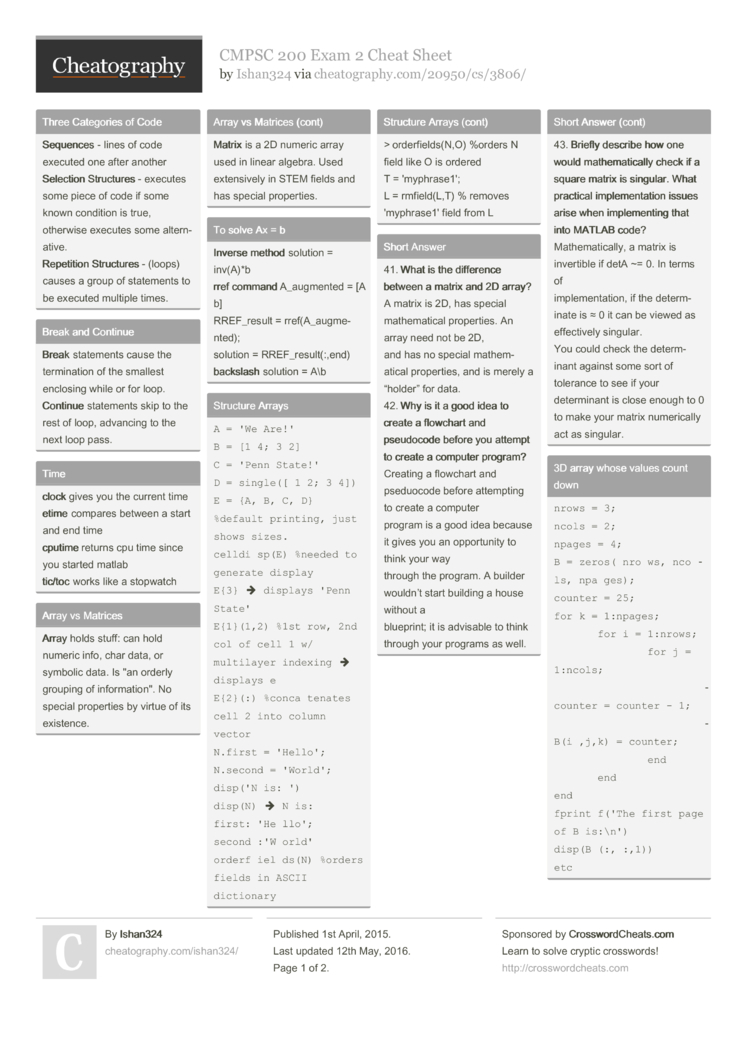# CMPSC 200 Exam 2 Cheat Sheet by Ishan324

### Three Categories of Code

 Sequ­ences - lines of code executed one after another Sele­ction Struct­ures - executes some piece of code if some known condition is true, otherwise executes some altern­ative. Repe­tition Struct­ures - (loops) causes a group of statements to be executed multiple times.

### Break and Continue

 Break statements cause the termin­ation of the smallest enclosing while or for loop. Cont­inue statements skip to the rest of loop, advancing to the next loop pass.

### Time

 clock gives you the current time etime compares between a start and end time cput­ime returns cpu time since you started matlab tic/­toc works like a stopwatch

### Array vs Matrices

 Array holds stuff: can hold numeric info, char data, or symbolic data. Is "an orderly grouping of inform­ati­on". No special properties by virtue of its existence. Matrix is a 2D numeric array used in linear algebra. Used extens­ively in STEM fields and has special proper­ties.

### To solve Ax = b

 Inverse method solution = inv(A)*b rref command A_augm­ented = [A b] RREF_r­esult = rref(A­_au­gme­nted); solution = RREF_r­esu­lt(­:,end) back­slash solution = A\b

### Structure Arrays

 ```A = 'We Are!' B = [1 4; 3 2] C = 'Penn State!' D = single([ 1 2; 3 4]) E = {A, B, C, D} %default printing, just shows sizes. celldisp(E) %needed to generate display E{3}  displays 'Penn State' E{1}(1,2) %1st row, 2nd col of cell 1 w/ multilayer indexing  displays e E{2}(:) %concatenates cell 2 into column vector N.first = 'Hello'; N.second = 'World'; disp('N is: ') disp(N)  N is: first:'Hello'; second:'World' orderfields(N) %orders fields in ASCII dictionary orderfields(N,O) %orders N field like O is ordered T = 'myphrase1'; L = rmfield(L,T) % removes 'myphrase1' field from L```

 41. What is the difference between a matrix and 2D array? A matrix is 2D, has special mathem­atical proper­ties. An array need not be 2D, and has no special mathem­atical proper­ties, and is merely a “holder” for data. 42. Why is it a good idea to create a flowchart and pseudocode before you attempt to create a computer program? Creating a flowchart and pseduocode before attempting to create a computer program is a good idea because it gives you an opport­unity to think your way through the program. A builder wouldn’t start building a house without a blueprint; it is advisable to think through your programs as well. 43. Briefly describe how one would mathem­ati­cally check if a square matrix is singular. What practical implem­ent­ation issues arise when implem­enting that into MATLAB code? Mathem­ati­cally, a matrix is invertible if detA ~= 0. In terms of implem­ent­ation, if the determ­inate is ≈ 0 it can be viewed as effect­ively singular. You could check the determ­inant against some sort of tolerance to see if your determ­inant is close enough to 0 to make your matrix numeri­cally act as singular.

### 3D array whose values count down

 ```nrows = 3; ncols = 2; npages = 4; B = zeros(nrows,ncols,npages); counter = 25; for k = 1:npages;     for i = 1:nrows;         for j = 1:ncols;             counter = counter - 1;             B(i,j,k) = counter;         end     end end fprintf('The first page of B is:\n') disp(B(:,:,1)) etc```

 44. What is the benefit of using cell arrays to store chars instead of using character arrays? Character arrays have to have the same number of columns in each row. Cell arrays of chars, however, has no such restri­ction. 45. Briefly discuss the difference between cell arrays and structure arrays in MATLAB. One of the more prominent differ­ences between cell arrays and structure arrays in MATLAB is content indexing for cells, and the use of fields for structure arrays.2 Pages
//media.cheatography.com/storage/thumb/ishan324_cmpsc-200-exam-2.750.jpg

PDF (recommended)